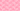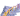PricingResources

# Calculating Present and Future Value of Annuity

Written by

Have you ever had to make a series of fixed payments over a set period of time? If so, you’re probably already familiar with the concept of annuities, even if you’re not so clued up on the terminology. Simply put, annuities are recurring or ongoing payments over a period of time, like rent or payments for a car. There are a couple of different ways that you can measure the cost or value of these annuities. Find out everything you need to know about calculating the present value of an annuity and the future value of an annuity with our helpful guide.

## Understanding the two types of annuities

Before we get started, let’s take a look at the two types of annuities. Essentially, there are ordinary annuities and annuities due. Ordinary annuities make/require payments at the end of each period, like bonds. By contrast, annuities due payments come at the beginning of each period, like rent. Now, we’ll explain how you can calculate the present and future values of these types of annuities.

## Calculating the present value of an annuity (ordinary and due)

Present value (PV) enables you to understand the present value of equally spaced payments in the future, provided a set interest rate. Use this annuity formula to calculate the present value of an ordinary annuity:

Where:

If you’d like to calculate the present value of an annuity due, you can use this annuity formula:

## Calculating the future value of an annuity (ordinary and due)

Essentially, future value (FV) measures the value of a series of payments at some point in the future, provided a specified interest rate. In other words, it’s the sum of the future value of each annuity payment. Fortunately, you don’t have to calculate each payment on an individual basis and add them all up. To calculate the future value of an ordinary annuity, you can use the following annuity formula:

• Future Value of an Ordinary Annuity = C x [(1+i)n – 1 / i)

If you want to calculate the future value of an annuity due, you can use this annuity formula:

• Future Value of an Annuity Due = C x [(1+i)n – 1 / i) x (1 + i)

At this point, it’s worth pointing out that r (interest rate) can’t be solved algebraically, it’s only ever going to be an estimate. There are a couple of different methods you can use to estimate r, including graphing calculators or plugging in different values for r with guesses. If you’re not too confident, you should contract this work to an accounting professional, as they’re best placed to handle these sorts of technical financial equations.

## Annuity calculators

Using the annuity formulas we’ve outlined above, it’s relatively easy to calculate the present value of an annuity and the future value of an annuity. However, there are a broad range of annuity calculators available online that you can use to calculate these sums for you, provided that you’ve got the correct inputs. This annuity calculator from CalculatorSoup is one such example, but there are plenty of others just a few clicks away if you don’t feel so confident handling the annuity formula yourself.

#### We can help

GoCardless helps you automate payment collection, cutting down on the amount of admin your team needs to deal with when chasing invoices. Find out how GoCardless can help you with ad hoc payments or recurring payments.## Interested in automating the way you get paid? GoCardless can help

Contact sales

Sales

Contact Sales

+1(415) 523-2279

Support

help@gocardless.com

+1 (628) 241-0044